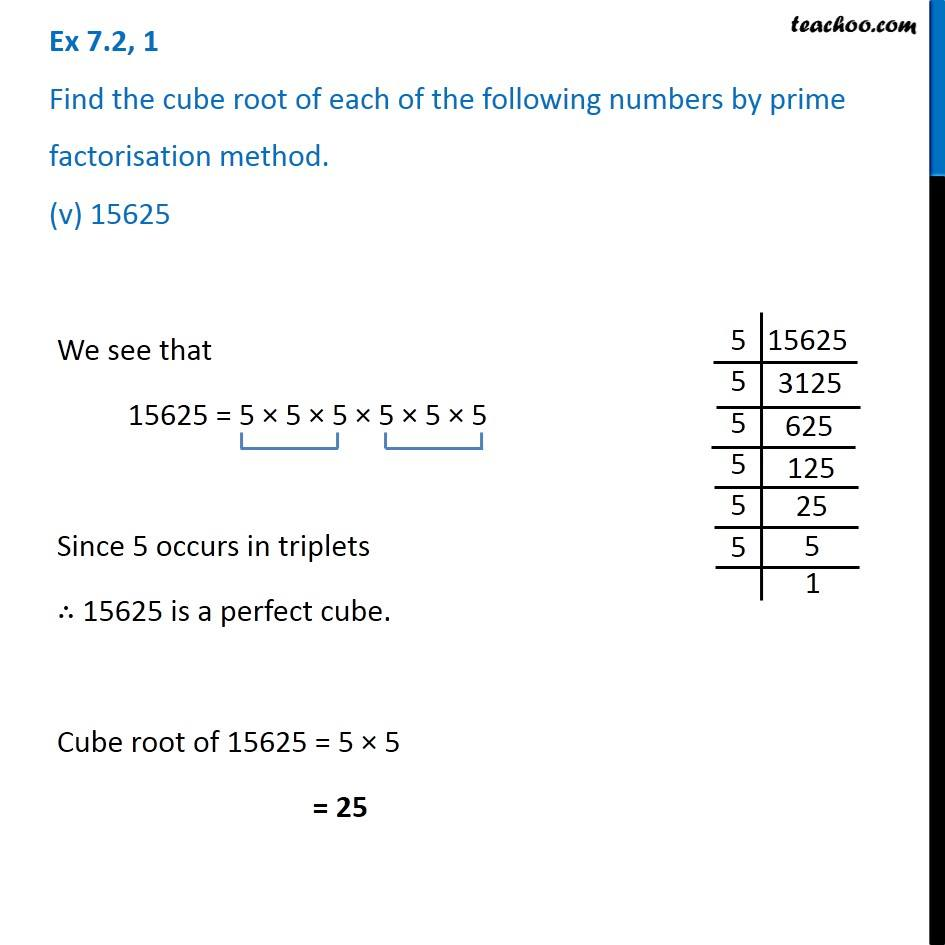1. Chapter 7 Class 8 Cubes and Cube Roots
2. Serial order wise
3. Ex 7.2

Transcript

Ex 7.2, 1 Find the cube root of each of the following numbers by prime factorisation method. (v) 15625We see that 15625 = 5 × 5 × 5 × 5 × 5 × 5 Since 5 occurs in triplets ∴ 15625 is a perfect cube. Cube root of 15625 = 5 × 5 = 25